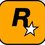Find the number of even numbers among the following 1001 numbers: $$\left( \begin{matrix} 1000 \\ 0 \end{matrix} \right) ,\left( \begin{matrix} 1000 \\ 1 \end{matrix} \right) ,\left( \begin{matrix} 1000 \\ 2 \end{matrix} \right) ,\left( \begin{matrix} 1000 \\ 3 \end{matrix} \right) ,\left( \begin{matrix} 1000 \\ 4 \end{matrix} \right) . . . \left( \begin{matrix} 1000 \\ 1000 \end{matrix} \right)$$.Note by Rohit Ner
6 years, 4 months ago

This discussion board is a place to discuss our Daily Challenges and the math and science related to those challenges. Explanations are more than just a solution — they should explain the steps and thinking strategies that you used to obtain the solution. Comments should further the discussion of math and science.

When posting on Brilliant:

• Use the emojis to react to an explanation, whether you're congratulating a job well done , or just really confused .
• Ask specific questions about the challenge or the steps in somebody's explanation. Well-posed questions can add a lot to the discussion, but posting "I don't understand!" doesn't help anyone.
• Try to contribute something new to the discussion, whether it is an extension, generalization or other idea related to the challenge.

MarkdownAppears as
*italics* or _italics_ italics
**bold** or __bold__ bold
- bulleted- list
• bulleted
• list
1. numbered2. list
1. numbered
2. list
Note: you must add a full line of space before and after lists for them to show up correctly
paragraph 1paragraph 2

paragraph 1

paragraph 2

[example link](https://brilliant.org)example link
> This is a quote
This is a quote
    # I indented these lines
# 4 spaces, and now they show
# up as a code block.

print "hello world"
# I indented these lines
# 4 spaces, and now they show
# up as a code block.

print "hello world"
MathAppears as
Remember to wrap math in $$ ... $$ or $ ... $ to ensure proper formatting.
2 \times 3 $2 \times 3$
2^{34} $2^{34}$
a_{i-1} $a_{i-1}$
\frac{2}{3} $\frac{2}{3}$
\sqrt{2} $\sqrt{2}$
\sum_{i=1}^3 $\sum_{i=1}^3$
\sin \theta $\sin \theta$
\boxed{123} $\boxed{123}$

Sort by:

It is probably easiest to use a consequence of Lucas's theorem. Since the base 2 expansion of $1000$ is $1111101000,$ those values $n$ between $0$ and $1000$ inclusive that have a $1$ in their base 2 expansion in at least one place where the expansion of $1000$ has a $0$ will render $\binom{1000}{m}$ even.

Now it will be easier to count the complement here, i.e., the number of values which have $0$'s in their binary expansion wherever the binary expansion of $1000$ does, and then subtract this from $1001.$ So after fixing the four $0$'s in place, we have six other digit positions which can be filled by either a $0$ or a $1.$ Thus there will be $2^{6} = 64$ values $m$ which will render $\binom{1000}{m}$ odd, and thus there are $1001 - 64 = 937$ numbers in the given list that are even.

- 6 years, 4 months ago

Thank you sir for posting such a beautiful approach! Please let me know if there is any alternative method as many jee aspirants are unaware of Lucas theorem. :)

- 6 years, 4 months ago

You're welcome. :) Yes, I noticed the JEE hashtag and wondered if this was a suitable approach for that exam, but I don't know of any other way of solving this problem without resorting to brute force. I'll be interested to see if anyone else knows of a JEE-friendly method.

- 6 years, 3 months ago

Can you help me finding the value of : $\huge\sum _{ r=0 }^{ n }{ \frac { { \left( -1 \right) }^{ r } }{ \left( \begin{matrix} n \\ r \end{matrix} \right) } }$

- 6 years, 3 months ago

First note that $\dbinom{n}{r} = \dbinom{n}{n - r}.$

Then if $n$ is odd, we would also have $(-1)^{r} = -((-1)^{n-r}),$ which would mean that all the terms in the sum would cancel pairwise, leaving us with an answer of $0.$

It gets more interesting if $n$ is even. I found formula #34 in this link, which is complicated, but with $p = 1$ it would appear to give a general solution of

$S_{n} = (1 + (-1)^{n})\dfrac{(n + 1)}{n + 2}.$

So for $n$ odd we have $S_{n} = 0$, (as found before), and for $n$ even we have $S_{n} = \dfrac{2(n + 1)}{n + 2}.$

I found another excellent resource here which confirms this result, (and much more).

Great question; I've never encountered this type of sum before. :)

- 6 years, 3 months ago

Thank you a lot for your help sir. :) .

- 6 years, 3 months ago

I posted a question inspired by this last question of yours. I hope you don't mind. :)

- 6 years, 3 months ago

Its a very good question sir! A pleasure to see a question inspired by me! :)

- 6 years, 3 months ago

I'm glad that you liked (and solved) it. :)

- 6 years, 3 months ago